# Welcome to the SolverTable add-in!!

This add-in is a natural extension to the Solver add-in incorporated in Excel. SolverTable performs sensitivity analysis for an Excel optimization model, and, in most cases, its output is more relevant and understandable than the optional sensitivity output provided by Solver itself. SolverTable is very easy to use, as described below. All it requires is an existing optimization model. That is, there must be a spreadsheet model already built, and the corresponding Solver dialog box must already have been filled out. In this help file, we will explain how to use SolverTable and illustrate it with several screen shots.

## Uninstalling SolverTable

To uninstall the add-in (remove it from memory), use Excel's Tools/Add-ins menu item and uncheck the SolverTable box.

## An example file

To illustrate SolverTable, we will use the standard product mix model, a version of which appears in Figure 1. (The conventions we use are that the inputs are in blue borders, the changing cells are in red borders, and the objective cell is in a black border.) We assume the Solver dialog box has already been completed in the obvious way (including checks in the Assume Linear Model and Assume Non-Negative boxes). Actually, this model has already been optimized, but this is not necessary for running SolverTable.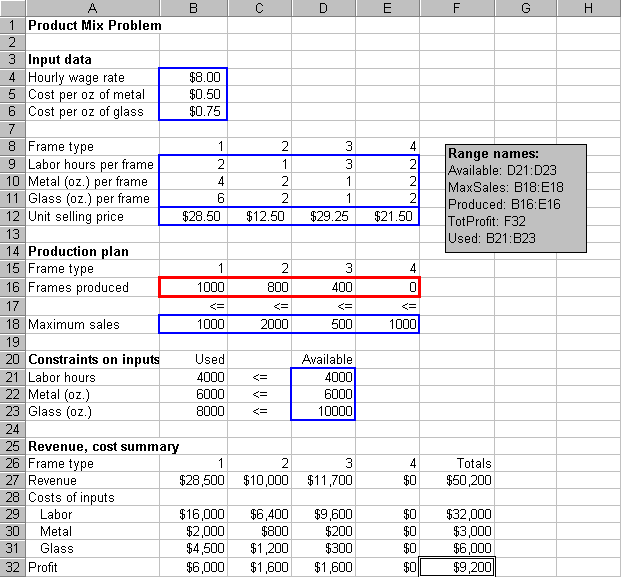Figure 1

## Running SolverTable

The purpose of SolverTable is very much like that of an Excel data table: to vary one or two inputs and keep track of one or more outputs. The main difference is that SolverTable runs Solver for each input value (or combination of input values) and reports the optimal results in the table. Like data tables, there are essentially two options: one-way tables and two-way tables, depending on whether there are one or two input cells. However, unlike Excel data tables, there can be multiple output cells for one-way and two-way tables. In the latter case, SolverTable creates a separate table for each output cell. Unfortunately (or fortunately, depending on your point of view), a table created by SolverTable retains no links to the original data. If you want to change anything about the table, you'll have to rerun SolverTable.

### Creating a one-way table

To illustrate a one-way table, we'll see how the optimal profit and the optimal product mix (changing cells) vary as the number of labor hours available (cell D21) varies from 2000 to 8000 in increments of 1000. Begin by selecting the Data/SolverTable menu item. This brings up the dialog box in Figure 2. Fill it out as shown and click on OK.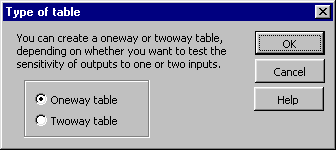Figure 2

The next dialog box, shown in Figure 3, requires you to specify the input cell, the input values (which are assumed to be in regular increments), the output cells, and a location for the table. Note that range names can be used if they exist. The add-in checks your entries in this dialog box for "obvious" errors. For example, the input cell should not contain a formula or a label, the output range should not contain the input cell, the table location shouldn't write over existing values (unless you say it is OK to do so), and so on. However, we won't guarantee that it checks for everything, so be careful when making these entries. Be especially careful that you choose a location for the table that doesn't write over any information you don't want to lose (even though you'll be warned if you try to do so).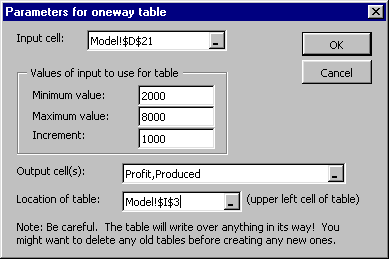Figure 3

The table itself appears in Figure 4. (We've added the heading in row 1 manually.) The small red triangles are Excel comments that SolverTable automatically adds to a number of cells. For example, the comment in cell I4 reminds what the input cell is (in this case, D21). The comments in column J are the Solver messages you normally see after running Solver. For this example, they all contain the message you hope to see: "Solver found a solution. All constraints and optimality conditions are satisfied." However, if there is no feasible solution, say, then the comment will alert you to this. Note that unlike an Excel data table, SolverTable does not put formulas at the top of the table (in row 3 of the figure), because this wouldn't make sense. However, it does put the cell addresses of the output cells. This way, you can remember which outputs are being reported.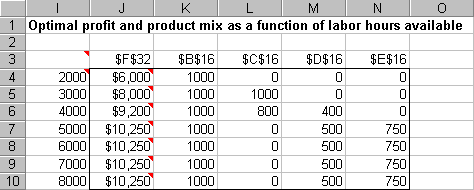Figure 4

### Creating a two-way table

To create a two-way table, go through the same steps as above for a one-way table, but check the two-way option in Figure 2. Then the two-way dialog box in Figure 5 appears. Here, we'll assume that both labor hour availability (D21) and hourly wage rate (B4) are being varied, and we want to keep track of the optimal profit and the number of labor hours used (B21). (Any output cells can be selected.)Figure 5

SolverTable then creates as many tables as there are output cells (in a vertical direction), as shown in Figure 6. Note that each cell in a table corresponds to a Solver run. For example, we see that when there are 4000 labor hours available and the wage rate is \$4 per hour, the optimal profit is \$25,200 (top table) and all 4000 labor hours are used (bottom table). Again, there are comments in selected cells. For example, the comments in cells I13 and J12 remind you that the input cells are D11 and B4. The comments in the body of each table report the Solver message, exactly as with one-way tables.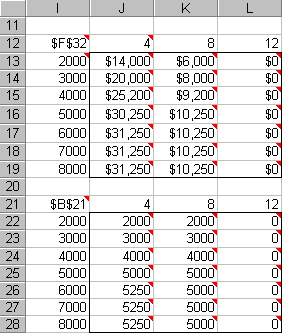Figure 6

As with all Solver models, it is a good idea to take a close look at the answers and see whether they make sense. For example, in Figure 6 we see all zeros in column L. Does this make sense? Yes, it probably does. When the labor rate is \$12 per hour and all other monetary inputs stay constant, labor is evidently too expensive for the company to make a profit. So it produces nothing!

### Being creative

With some imagination, you can get SolverTable to run some really interesting sensitivity analyses. For example, suppose we want to allow the availabilities of all three resources (labor, metal, and glass) to change by common factor. Then we need to change the model slightly, as indicated in Figure 7. Now the original availabilities have been moved to column F, a change factor has been inserted in column H, and formulas have been entered for availabilities in column D. Specifically, the formula in cell D21 is =\$H\$21*F21, which is then copied down.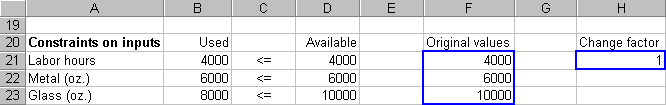Figure 7

We can now do a one-way sensitivity analysis on this change factor, using the settings in Figure 8. The corresponding table appears in Figure 9.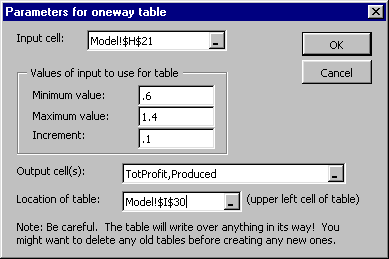Figure 8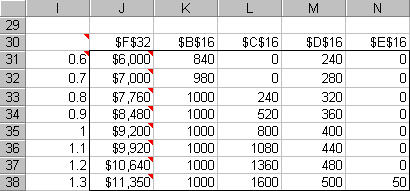Figure 9

As another example, suppose you want to keep track of a function of several cells in the model. For example, suppose you want to keep track of the maximum number of frames of any single type produced, that is, the maximum of the changing cell values. You can't select this as an output directly because it doesn't appear anywhere in the model. But the solution is simple: Create a formula for it in some unused cell, and then specify this cell as an output cell.

## SolverTable Difficulties

SolverTable relies on Solver. In fact, SolverTable invokes Solver for each input value (or combination of input values). Therefore, all of the problems that people have experienced with Solver are liable to occur with SolverTable. However, we have tried to overcome one of Solver's more annoying features: claiming that a model is not linear when we know it is linear. In case you haven't run into this problem, we'll describe it first. When your model is linear, you should check the Assume Linear Model box under Solver Options. This has two advantages. First, it uses a faster algorithm (the simplex method). Second, it is guaranteed (well, almost) to find the optimal solution if an optimal solution exists. However, Solver uses its own rules to check whether a model is linear. Because of numerical precision problems inherent in computers, Solver sometimes decides that a model is not linear, even though we know it is. In this case, it responds with an error message that the conditions for a linear model are not satisfied.

SolverTable automatically checks for this error message. If Solver gives it, then SolverTable reruns Solver (for this problem) with the Assume Linear Model box unchecked and reports the result. However, to let you know that all of this happened, the corresponding cells of the table are colored yellow, and the comments in these cells describe the problem. An example we encountered appears in Figure 10. This model is indeed linear. However, when we formed the one-way table, Solver thought the model was not linear for input values of 0.80 and 1.00, even though it agreed that it was linear for input values of 0.90 and 1.10. Similarly, for the two-way table, it thought the model was not linear for 2 of the 16 input combinations. This is clearly strange behavior, but it happens fairly frequently. At least, SolverTable provides a solution (using a nonlinear algorithm) rather than the annoying "conditions for linear model are not satisfied" Solver message.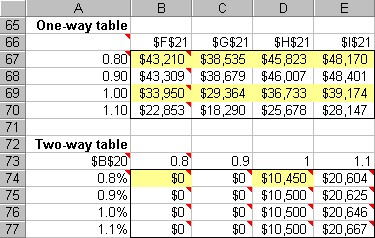Figure 10